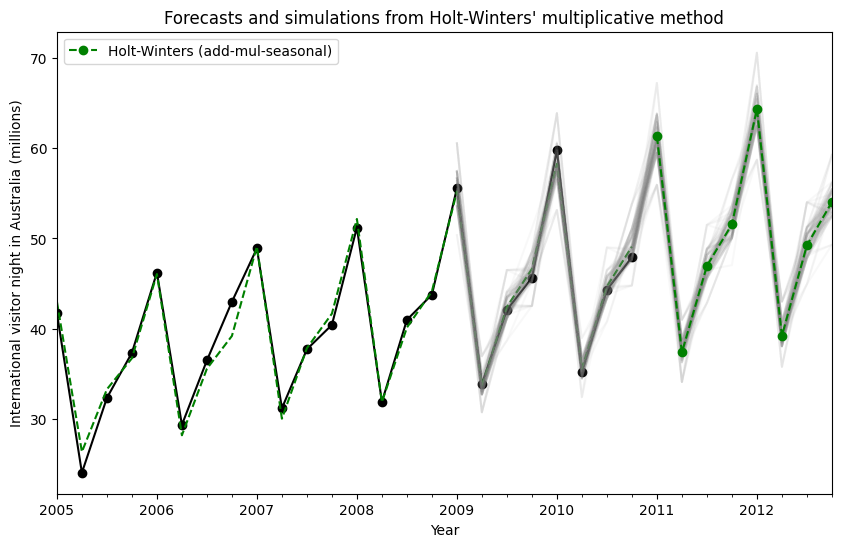# Exponential smoothing¶

Let us consider chapter 7 of the excellent treatise on the subject of Exponential Smoothing By Hyndman and Athanasopoulos . We will work through all the examples in the chapter as they unfold.

First we load some data. We have included the R data in the notebook for expedience.

:

import os
import numpy as np
import pandas as pd
import matplotlib.pyplot as plt
from statsmodels.tsa.api import ExponentialSmoothing, SimpleExpSmoothing, Holt

%matplotlib inline

data = [
446.6565,
454.4733,
455.663,
423.6322,
456.2713,
440.5881,
425.3325,
485.1494,
506.0482,
526.792,
514.2689,
494.211,
]
index = pd.date_range(start="1996", end="2008", freq="A")
oildata = pd.Series(data, index)

data = [
17.5534,
21.86,
23.8866,
26.9293,
26.8885,
28.8314,
30.0751,
30.9535,
30.1857,
31.5797,
32.5776,
33.4774,
39.0216,
41.3864,
41.5966,
]
index = pd.date_range(start="1990", end="2005", freq="A")
air = pd.Series(data, index)

data = [
263.9177,
268.3072,
260.6626,
266.6394,
277.5158,
283.834,
290.309,
292.4742,
300.8307,
309.2867,
318.3311,
329.3724,
338.884,
339.2441,
328.6006,
314.2554,
314.4597,
321.4138,
329.7893,
346.3852,
352.2979,
348.3705,
417.5629,
417.1236,
417.7495,
412.2339,
411.9468,
394.6971,
401.4993,
408.2705,
414.2428,
]
index = pd.date_range(start="1970", end="2001", freq="A")
livestock2 = pd.Series(data, index)

data = [407.9979, 403.4608, 413.8249, 428.105, 445.3387, 452.9942, 455.7402]
index = pd.date_range(start="2001", end="2008", freq="A")
livestock3 = pd.Series(data, index)

data = [
41.7275,
24.0418,
32.3281,
37.3287,
46.2132,
29.3463,
36.4829,
42.9777,
48.9015,
31.1802,
37.7179,
40.4202,
51.2069,
31.8872,
40.9783,
43.7725,
55.5586,
33.8509,
42.0764,
45.6423,
59.7668,
35.1919,
44.3197,
47.9137,
]
index = pd.date_range(start="2005", end="2010-Q4", freq="QS-OCT")
aust = pd.Series(data, index)


## Simple Exponential Smoothing¶

Lets use Simple Exponential Smoothing to forecast the below oil data.

:

ax = oildata.plot()
ax.set_xlabel("Year")
ax.set_ylabel("Oil (millions of tonnes)")
print("Figure 7.1: Oil production in Saudi Arabia from 1996 to 2007.")

Figure 7.1: Oil production in Saudi Arabia from 1996 to 2007.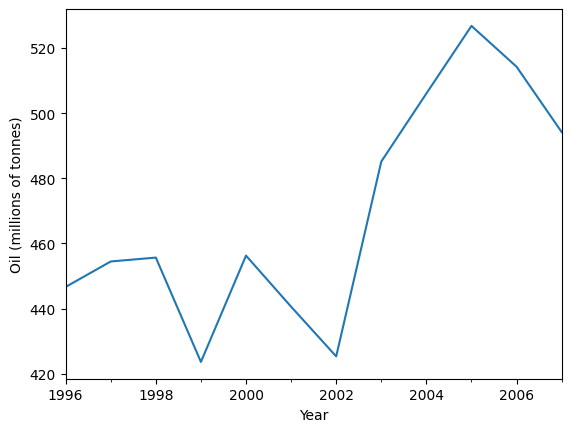Here we run three variants of simple exponential smoothing: 1. In fit1 we do not use the auto optimization but instead choose to explicitly provide the model with the $$\alpha=0.2$$ parameter 2. In fit2 as above we choose an $$\alpha=0.6$$ 3. In fit3 we allow statsmodels to automatically find an optimized $$\alpha$$ value for us. This is the recommended approach.

:

fit1 = SimpleExpSmoothing(oildata, initialization_method="heuristic").fit(
smoothing_level=0.2, optimized=False
)
fcast1 = fit1.forecast(3).rename(r"$\alpha=0.2$")
fit2 = SimpleExpSmoothing(oildata, initialization_method="heuristic").fit(
smoothing_level=0.6, optimized=False
)
fcast2 = fit2.forecast(3).rename(r"$\alpha=0.6$")
fit3 = SimpleExpSmoothing(oildata, initialization_method="estimated").fit()
fcast3 = fit3.forecast(3).rename(r"$\alpha=%s$" % fit3.model.params["smoothing_level"])

plt.figure(figsize=(12, 8))
plt.plot(oildata, marker="o", color="black")
plt.plot(fit1.fittedvalues, marker="o", color="blue")
(line1,) = plt.plot(fcast1, marker="o", color="blue")
plt.plot(fit2.fittedvalues, marker="o", color="red")
(line2,) = plt.plot(fcast2, marker="o", color="red")
plt.plot(fit3.fittedvalues, marker="o", color="green")
(line3,) = plt.plot(fcast3, marker="o", color="green")
plt.legend([line1, line2, line3], [fcast1.name, fcast2.name, fcast3.name])

:

<matplotlib.legend.Legend at 0x7f9e88025990>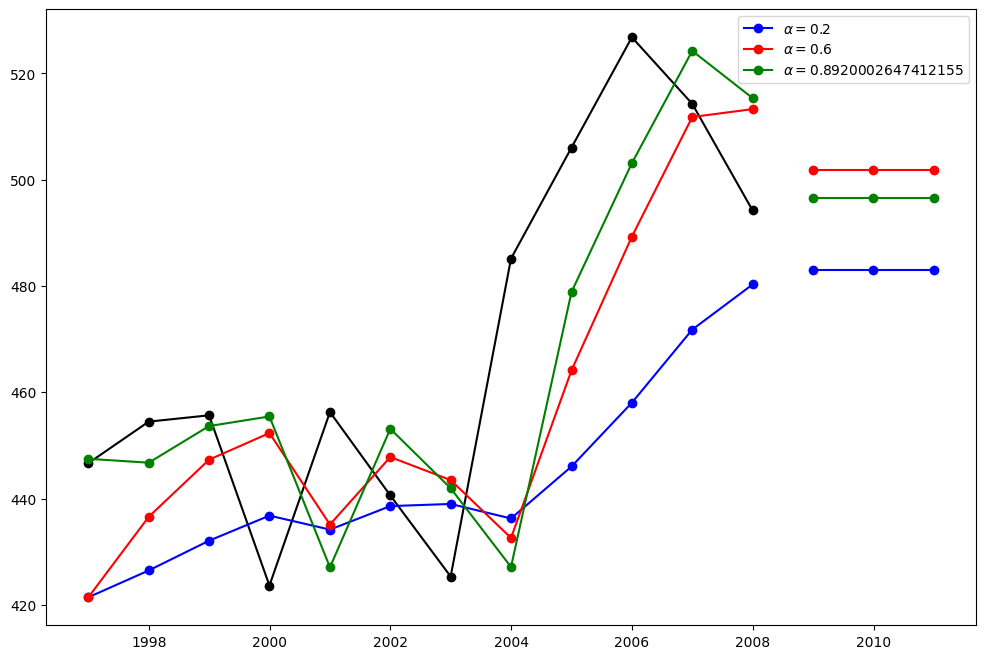## Holt’s Method¶

Lets take a look at another example. This time we use air pollution data and the Holt’s Method. We will fit three examples again. 1. In fit1 we again choose not to use the optimizer and provide explicit values for $$\alpha=0.8$$ and $$\beta=0.2$$ 2. In fit2 we do the same as in fit1 but choose to use an exponential model rather than a Holt’s additive model. 3. In fit3 we used a damped versions of the Holt’s additive model but allow the dampening parameter $$\phi$$ to be optimized while fixing the values for $$\alpha=0.8$$ and $$\beta=0.2$$

:

fit1 = Holt(air, initialization_method="estimated").fit(
smoothing_level=0.8, smoothing_trend=0.2, optimized=False
)
fcast1 = fit1.forecast(5).rename("Holt's linear trend")
fit2 = Holt(air, exponential=True, initialization_method="estimated").fit(
smoothing_level=0.8, smoothing_trend=0.2, optimized=False
)
fcast2 = fit2.forecast(5).rename("Exponential trend")
fit3 = Holt(air, damped_trend=True, initialization_method="estimated").fit(
smoothing_level=0.8, smoothing_trend=0.2
)

plt.figure(figsize=(12, 8))
plt.plot(air, marker="o", color="black")
plt.plot(fit1.fittedvalues, color="blue")
(line1,) = plt.plot(fcast1, marker="o", color="blue")
plt.plot(fit2.fittedvalues, color="red")
(line2,) = plt.plot(fcast2, marker="o", color="red")
plt.plot(fit3.fittedvalues, color="green")
(line3,) = plt.plot(fcast3, marker="o", color="green")
plt.legend([line1, line2, line3], [fcast1.name, fcast2.name, fcast3.name])

:

<matplotlib.legend.Legend at 0x7f9e87c35810>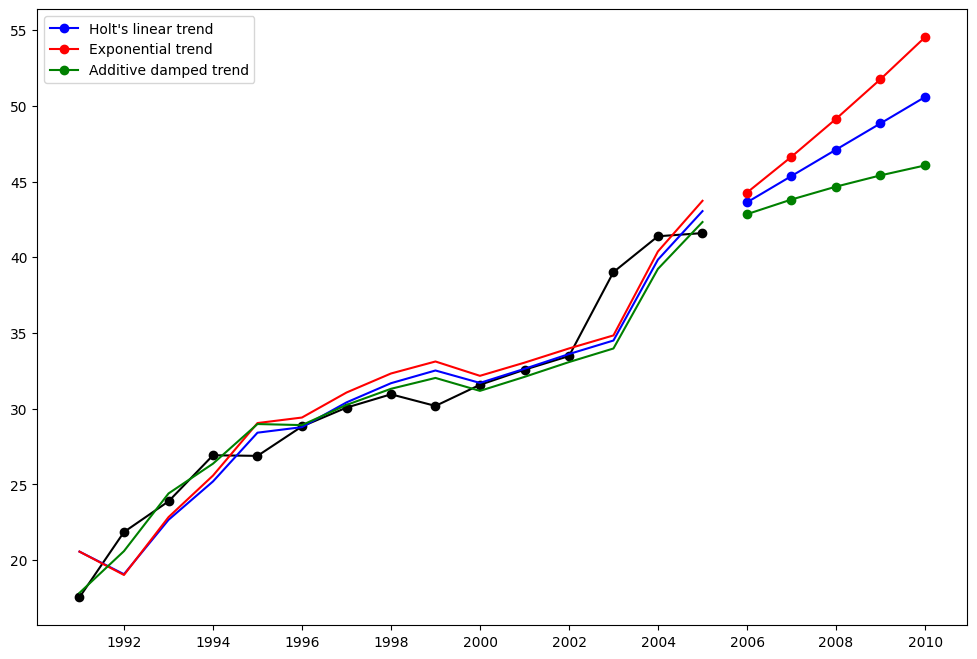Lets look at some seasonally adjusted livestock data. We fit five Holt’s models. The below table allows us to compare results when we use exponential versus additive and damped versus non-damped.

Note: fit4 does not allow the parameter $$\phi$$ to be optimized by providing a fixed value of $$\phi=0.98$$

:

fit1 = SimpleExpSmoothing(livestock2, initialization_method="estimated").fit()
fit2 = Holt(livestock2, initialization_method="estimated").fit()
fit3 = Holt(livestock2, exponential=True, initialization_method="estimated").fit()
fit4 = Holt(livestock2, damped_trend=True, initialization_method="estimated").fit(
damping_trend=0.98
)
fit5 = Holt(
livestock2, exponential=True, damped_trend=True, initialization_method="estimated"
).fit()
params = [
"smoothing_level",
"smoothing_trend",
"damping_trend",
"initial_level",
"initial_trend",
]
results = pd.DataFrame(
index=[r"$\alpha$", r"$\beta$", r"$\phi$", r"$l_0$", "$b_0$", "SSE"],
)
results["SES"] = [fit1.params[p] for p in params] + [fit1.sse]
results["Holt's"] = [fit2.params[p] for p in params] + [fit2.sse]
results["Exponential"] = [fit3.params[p] for p in params] + [fit3.sse]
results["Additive"] = [fit4.params[p] for p in params] + [fit4.sse]
results["Multiplicative"] = [fit5.params[p] for p in params] + [fit5.sse]
results

:

$\alpha$ 1.000000 0.974308 9.776329e-01 0.978852 0.974891
$\beta$ NaN 0.000000 4.016578e-12 0.000000 0.000000
$\phi$ NaN NaN NaN 0.980000 0.981637
$l_0$ 263.917688 258.882600 2.603440e+02 257.357526 258.940454
$b_0$ NaN 5.010783 1.013780e+00 6.644741 1.038159
SSE 6761.350235 6004.138200 6.104195e+03 6036.555005 6081.995166

### Plots of Seasonally Adjusted Data¶

The following plots allow us to evaluate the level and slope/trend components of the above table’s fits.

:

for fit in [fit2, fit4]:
pd.DataFrame(np.c_[fit.level, fit.trend]).rename(
columns={0: "level", 1: "slope"}
).plot(subplots=True)
plt.show()
print(
"Figure 7.4: Level and slope components for Holt’s linear trend method and the additive damped trend method."
)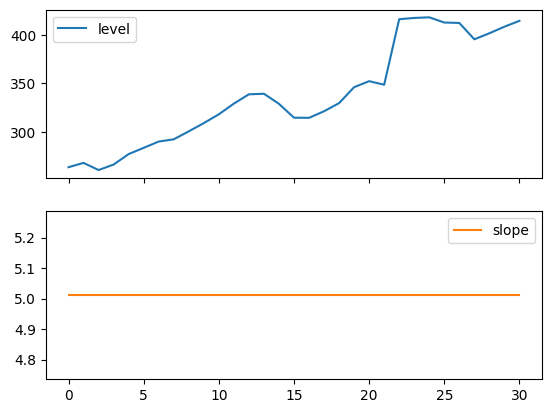Figure 7.4: Level and slope components for Holt’s linear trend method and the additive damped trend method.


## Comparison¶

Here we plot a comparison Simple Exponential Smoothing and Holt’s Methods for various additive, exponential and damped combinations. All of the models parameters will be optimized by statsmodels.

:

fit1 = SimpleExpSmoothing(livestock2, initialization_method="estimated").fit()
fcast1 = fit1.forecast(9).rename("SES")
fit2 = Holt(livestock2, initialization_method="estimated").fit()
fcast2 = fit2.forecast(9).rename("Holt's")
fit3 = Holt(livestock2, exponential=True, initialization_method="estimated").fit()
fcast3 = fit3.forecast(9).rename("Exponential")
fit4 = Holt(livestock2, damped_trend=True, initialization_method="estimated").fit(
damping_trend=0.98
)
fit5 = Holt(
livestock2, exponential=True, damped_trend=True, initialization_method="estimated"
).fit()
fcast5 = fit5.forecast(9).rename("Multiplicative Damped")

ax = livestock2.plot(color="black", marker="o", figsize=(12, 8))
livestock3.plot(ax=ax, color="black", marker="o", legend=False)
fcast1.plot(ax=ax, color="red", legend=True)
fcast2.plot(ax=ax, color="green", legend=True)
fcast3.plot(ax=ax, color="blue", legend=True)
fcast4.plot(ax=ax, color="cyan", legend=True)
fcast5.plot(ax=ax, color="magenta", legend=True)
ax.set_ylabel("Livestock, sheep in Asia (millions)")
plt.show()
print(
"Figure 7.5: Forecasting livestock, sheep in Asia: comparing forecasting performance of non-seasonal methods."
)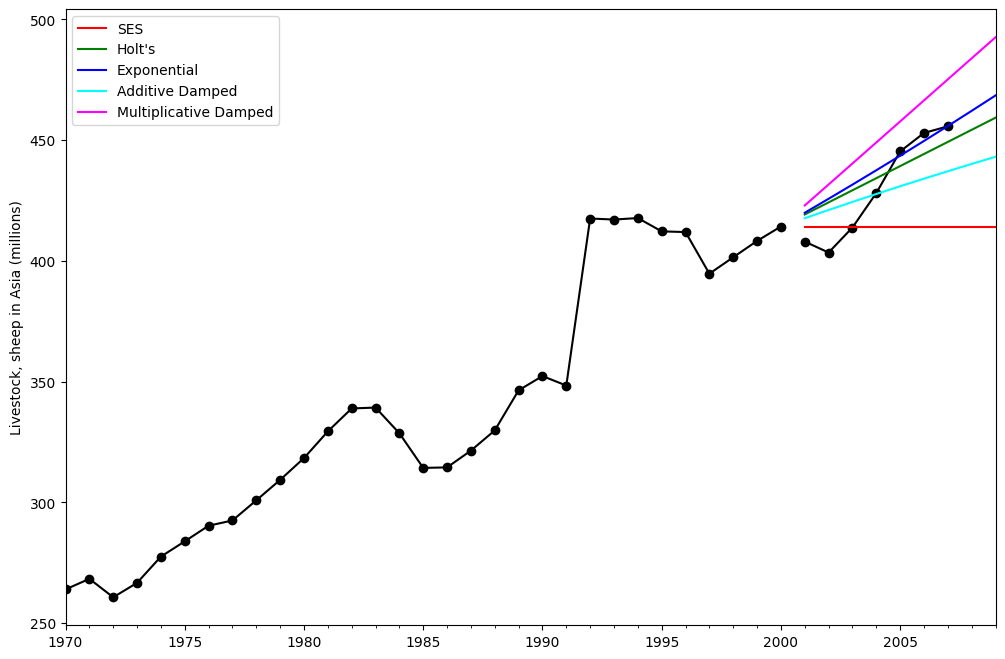Figure 7.5: Forecasting livestock, sheep in Asia: comparing forecasting performance of non-seasonal methods.


## Holt’s Winters Seasonal¶

Finally we are able to run full Holt’s Winters Seasonal Exponential Smoothing including a trend component and a seasonal component. statsmodels allows for all the combinations including as shown in the examples below: 1. fit1 additive trend, additive seasonal of period season_length=4 and the use of a Box-Cox transformation. 1. fit2 additive trend, multiplicative seasonal of period season_length=4 and the use of a Box-Cox transformation.. 1. fit3 additive damped trend, additive seasonal of period season_length=4 and the use of a Box-Cox transformation. 1. fit4 additive damped trend, multiplicative seasonal of period season_length=4 and the use of a Box-Cox transformation.

The plot shows the results and forecast for fit1 and fit2. The table allows us to compare the results and parameterizations.

:

fit1 = ExponentialSmoothing(
aust,
seasonal_periods=4,
use_boxcox=True,
initialization_method="estimated",
).fit()
fit2 = ExponentialSmoothing(
aust,
seasonal_periods=4,
seasonal="mul",
use_boxcox=True,
initialization_method="estimated",
).fit()
fit3 = ExponentialSmoothing(
aust,
seasonal_periods=4,
damped_trend=True,
use_boxcox=True,
initialization_method="estimated",
).fit()
fit4 = ExponentialSmoothing(
aust,
seasonal_periods=4,
seasonal="mul",
damped_trend=True,
use_boxcox=True,
initialization_method="estimated",
).fit()
results = pd.DataFrame(
index=[r"$\alpha$", r"$\beta$", r"$\phi$", r"$\gamma$", r"$l_0$", "$b_0$", "SSE"]
)
params = [
"smoothing_level",
"smoothing_trend",
"damping_trend",
"smoothing_seasonal",
"initial_level",
"initial_trend",
]
results["Additive"] = [fit1.params[p] for p in params] + [fit1.sse]
results["Multiplicative"] = [fit2.params[p] for p in params] + [fit2.sse]
results["Additive Dam"] = [fit3.params[p] for p in params] + [fit3.sse]
results["Multiplica Dam"] = [fit4.params[p] for p in params] + [fit4.sse]

ax = aust.plot(
figsize=(10, 6),
marker="o",
color="black",
title="Forecasts from Holt-Winters' multiplicative method",
)
ax.set_ylabel("International visitor night in Australia (millions)")
ax.set_xlabel("Year")
fit1.fittedvalues.plot(ax=ax, style="--", color="red")
fit2.fittedvalues.plot(ax=ax, style="--", color="green")

ax=ax, style="--", marker="o", color="red", legend=True
)
ax=ax, style="--", marker="o", color="green", legend=True
)

plt.show()
print(
"Figure 7.6: Forecasting international visitor nights in Australia using Holt-Winters method with both additive and multiplicative seasonality."
)

results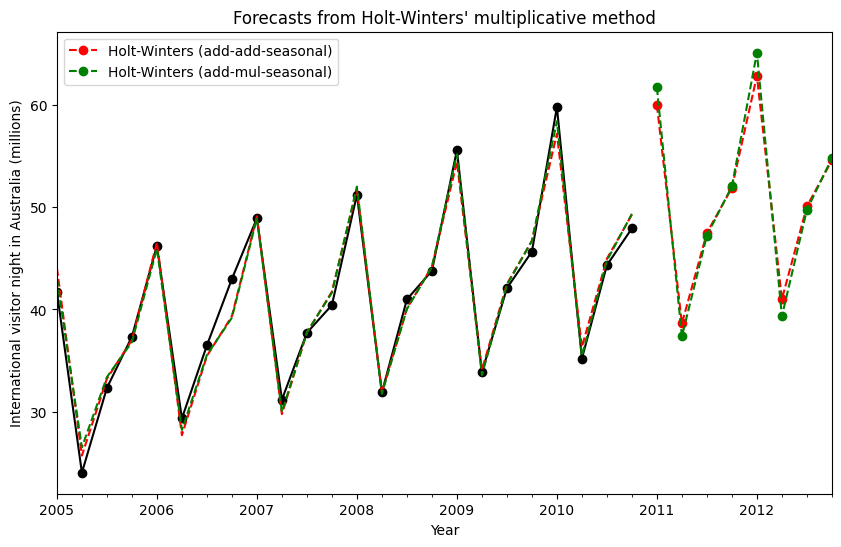Figure 7.6: Forecasting international visitor nights in Australia using Holt-Winters method with both additive and multiplicative seasonality.

:

$\alpha$ 1.490116e-08 1.490116e-08 1.490116e-08 1.490116e-08
$\beta$ 1.409865e-08 0.000000e+00 6.490761e-09 5.042250e-09
$\phi$ NaN NaN 9.430416e-01 9.536043e-01
$\gamma$ 7.544975e-16 1.889367e-15 7.434023e-16 1.273086e-16
$l_0$ 1.119348e+01 1.106376e+01 1.084022e+01 9.899296e+00
$b_0$ 1.205396e-01 1.198957e-01 2.456749e-01 1.975447e-01
SSE 4.402746e+01 3.611262e+01 3.527620e+01 3.062033e+01

### The Internals¶

It is possible to get at the internals of the Exponential Smoothing models.

Here we show some tables that allow you to view side by side the original values $$y_t$$, the level $$l_t$$, the trend $$b_t$$, the season $$s_t$$ and the fitted values $$\hat{y}_t$$. Note that these values only have meaningful values in the space of your original data if the fit is performed without a Box-Cox transformation.

:

fit1 = ExponentialSmoothing(
aust,
seasonal_periods=4,
initialization_method="estimated",
).fit()
fit2 = ExponentialSmoothing(
aust,
seasonal_periods=4,
seasonal="mul",
initialization_method="estimated",
).fit()

:

df = pd.DataFrame(
np.c_[aust, fit1.level, fit1.trend, fit1.season, fit1.fittedvalues],
columns=[r"$y_t$", r"$l_t$", r"$b_t$", r"$s_t$", r"$\hat{y}_t$"],
index=aust.index,
)
df.append(fit1.forecast(8).rename(r"$\hat{y}_t$").to_frame(), sort=True)

/tmp/ipykernel_5494/776371549.py:6: FutureWarning: The frame.append method is deprecated and will be removed from pandas in a future version. Use pandas.concat instead.
df.append(fit1.forecast(8).rename(r"$\hat{y}_t$").to_frame(), sort=True)

:

$\hat{y}_t$ $b_t$ $l_t$ $s_t$ $y_t$
2005-01-01 44.584128 0.597822 34.297580 10.286548 41.7275
2005-04-01 24.938189 0.597822 34.895402 -9.957213 24.0418
2005-07-01 33.005765 0.597822 35.493224 -2.487458 32.3281
2005-10-01 37.031107 0.597822 36.091046 0.940062 37.3287
2006-01-01 46.975415 0.597822 36.688868 10.286548 46.2132
2006-04-01 27.329477 0.597822 37.286690 -9.957213 29.3463
2006-07-01 35.397053 0.597822 37.884512 -2.487458 36.4829
2006-10-01 39.422395 0.597822 38.482334 0.940062 42.9777
2007-01-01 49.366703 0.597822 39.080156 10.286548 48.9015
2007-04-01 29.720765 0.597822 39.677978 -9.957213 31.1802
2007-07-01 37.788341 0.597822 40.275800 -2.487458 37.7179
2007-10-01 41.813683 0.597822 40.873622 0.940062 40.4202
2008-01-01 51.757991 0.597822 41.471444 10.286548 51.2069
2008-04-01 32.112053 0.597822 42.069266 -9.957213 31.8872
2008-07-01 40.179629 0.597822 42.667088 -2.487458 40.9783
2008-10-01 44.204971 0.597822 43.264910 0.940062 43.7725
2009-01-01 54.149279 0.597822 43.862732 10.286548 55.5586
2009-04-01 34.503341 0.597822 44.460554 -9.957213 33.8509
2009-07-01 42.570917 0.597822 45.058376 -2.487458 42.0764
2009-10-01 46.596259 0.597822 45.656197 0.940062 45.6423
2010-01-01 56.540567 0.597822 46.254020 10.286548 59.7668
2010-04-01 36.894628 0.597822 46.851841 -9.957213 35.1919
2010-07-01 44.962205 0.597822 47.449663 -2.487458 44.3197
2010-10-01 48.987547 0.597822 48.047485 0.940062 47.9137
2011-01-01 58.931855 NaN NaN NaN NaN
2011-04-01 39.285916 NaN NaN NaN NaN
2011-07-01 47.353493 NaN NaN NaN NaN
2011-10-01 51.378835 NaN NaN NaN NaN
2012-01-01 61.323143 NaN NaN NaN NaN
2012-04-01 41.677204 NaN NaN NaN NaN
2012-07-01 49.744781 NaN NaN NaN NaN
2012-10-01 53.770123 NaN NaN NaN NaN
:

df = pd.DataFrame(
np.c_[aust, fit2.level, fit2.trend, fit2.season, fit2.fittedvalues],
columns=[r"$y_t$", r"$l_t$", r"$b_t$", r"$s_t$", r"$\hat{y}_t$"],
index=aust.index,
)
df.append(fit2.forecast(8).rename(r"$\hat{y}_t$").to_frame(), sort=True)

/tmp/ipykernel_5494/3145826131.py:6: FutureWarning: The frame.append method is deprecated and will be removed from pandas in a future version. Use pandas.concat instead.
df.append(fit2.forecast(8).rename(r"$\hat{y}_t$").to_frame(), sort=True)

:

$\hat{y}_t$ $b_t$ $l_t$ $s_t$ $y_t$
2005-01-01 43.005388 0.620934 35.016002 1.228164 41.7275
2005-04-01 26.352949 0.620934 35.636936 0.739484 24.0418
2005-07-01 33.284728 0.620934 36.257870 0.918000 32.3281
2005-10-01 36.719508 0.620934 36.878804 0.995681 37.3287
2006-01-01 46.055823 0.620934 37.499738 1.228164 46.2132
2006-04-01 28.189633 0.620934 38.120672 0.739484 29.3463
2006-07-01 35.564799 0.620934 38.741606 0.918000 36.4829
2006-10-01 39.192516 0.620934 39.362540 0.995681 42.9777
2007-01-01 49.106258 0.620934 39.983474 1.228164 48.9015
2007-04-01 30.026316 0.620934 40.604408 0.739484 31.1802
2007-07-01 37.844869 0.620934 41.225342 0.918000 37.7179
2007-10-01 41.665524 0.620934 41.846277 0.995681 40.4202
2008-01-01 52.156693 0.620934 42.467211 1.228164 51.2069
2008-04-01 31.863000 0.620934 43.088145 0.739484 31.8872
2008-07-01 40.124939 0.620934 43.709079 0.918000 40.9783
2008-10-01 44.138531 0.620934 44.330013 0.995681 43.7725
2009-01-01 55.207128 0.620934 44.950947 1.228164 55.5586
2009-04-01 33.699683 0.620934 45.571881 0.739484 33.8509
2009-07-01 42.405009 0.620934 46.192815 0.918000 42.0764
2009-10-01 46.611539 0.620934 46.813749 0.995681 45.6423
2010-01-01 58.257563 0.620934 47.434683 1.228164 59.7668
2010-04-01 35.536367 0.620934 48.055617 0.739484 35.1919
2010-07-01 44.685079 0.620934 48.676551 0.918000 44.3197
2010-10-01 49.084547 0.620934 49.297485 0.995681 47.9137
2011-01-01 61.307998 NaN NaN NaN NaN
2011-04-01 37.373051 NaN NaN NaN NaN
2011-07-01 46.965149 NaN NaN NaN NaN
2011-10-01 51.557555 NaN NaN NaN NaN
2012-01-01 64.358434 NaN NaN NaN NaN
2012-04-01 39.209734 NaN NaN NaN NaN
2012-07-01 49.245220 NaN NaN NaN NaN
2012-10-01 54.030563 NaN NaN NaN NaN

Finally lets look at the levels, slopes/trends and seasonal components of the models.

:

states1 = pd.DataFrame(
np.c_[fit1.level, fit1.trend, fit1.season],
columns=["level", "slope", "seasonal"],
index=aust.index,
)
states2 = pd.DataFrame(
np.c_[fit2.level, fit2.trend, fit2.season],
columns=["level", "slope", "seasonal"],
index=aust.index,
)
fig, [[ax1, ax4], [ax2, ax5], [ax3, ax6]] = plt.subplots(3, 2, figsize=(12, 8))
states1[["level"]].plot(ax=ax1)
states1[["slope"]].plot(ax=ax2)
states1[["seasonal"]].plot(ax=ax3)
states2[["level"]].plot(ax=ax4)
states2[["slope"]].plot(ax=ax5)
states2[["seasonal"]].plot(ax=ax6)
plt.show()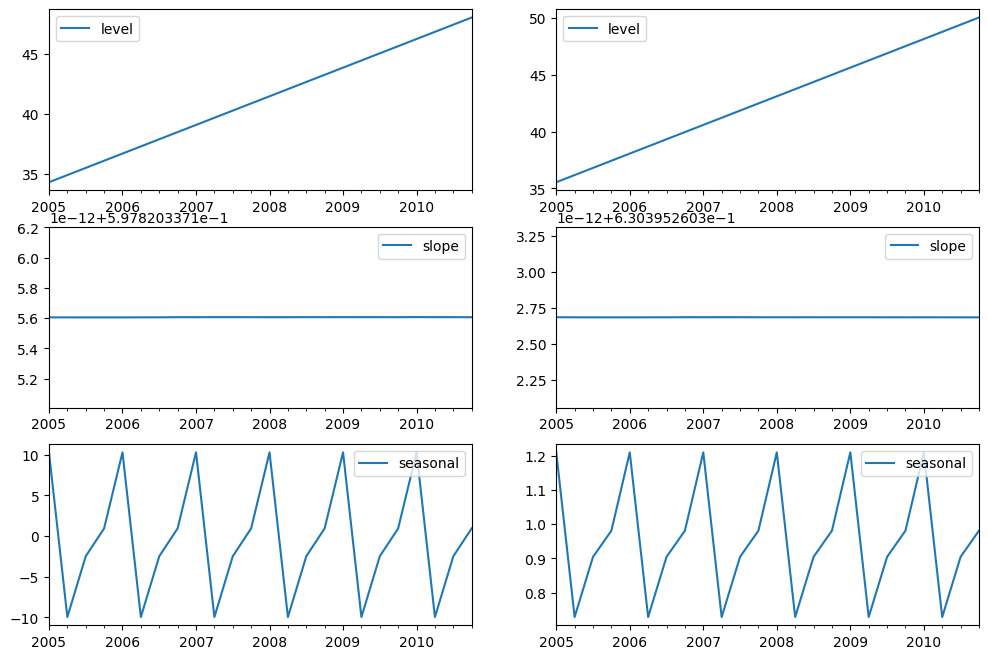# Simulations and Confidence Intervals¶

By using a state space formulation, we can perform simulations of future values. The mathematical details are described in Hyndman and Athanasopoulos  and in the documentation of HoltWintersResults.simulate.

Similar to the example in , we use the model with additive trend, multiplicative seasonality, and multiplicative error. We simulate up to 8 steps into the future, and perform 1000 simulations. As can be seen in the below figure, the simulations match the forecast values quite well.

:

fit = ExponentialSmoothing(
aust,
seasonal_periods=4,
seasonal="mul",
initialization_method="estimated",
).fit()
simulations = fit.simulate(8, repetitions=100, error="mul")

ax = aust.plot(
figsize=(10, 6),
marker="o",
color="black",
title="Forecasts and simulations from Holt-Winters' multiplicative method",
)
ax.set_ylabel("International visitor night in Australia (millions)")
ax.set_xlabel("Year")
fit.fittedvalues.plot(ax=ax, style="--", color="green")
simulations.plot(ax=ax, style="-", alpha=0.05, color="grey", legend=False)
ax=ax, style="--", marker="o", color="green", legend=True
)
plt.show()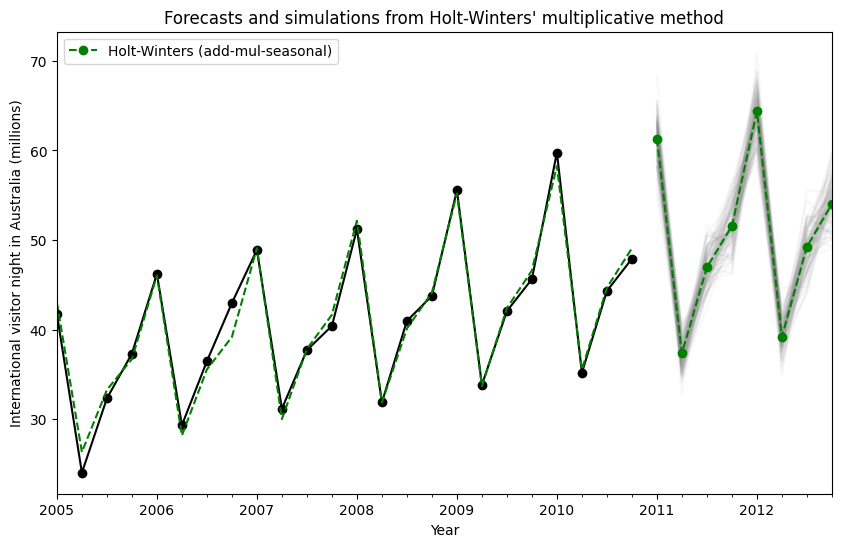Simulations can also be started at different points in time, and there are multiple options for choosing the random noise.

:

fit = ExponentialSmoothing(
aust,
seasonal_periods=4,
seasonal="mul",
initialization_method="estimated",
).fit()
simulations = fit.simulate(
16, anchor="2009-01-01", repetitions=100, error="mul", random_errors="bootstrap"
)

ax = aust.plot(
figsize=(10, 6),
marker="o",
color="black",
title="Forecasts and simulations from Holt-Winters' multiplicative method",
)
ax.set_ylabel("International visitor night in Australia (millions)")
ax.set_xlabel("Year")
fit.fittedvalues.plot(ax=ax, style="--", color="green")
simulations.plot(ax=ax, style="-", alpha=0.05, color="grey", legend=False)